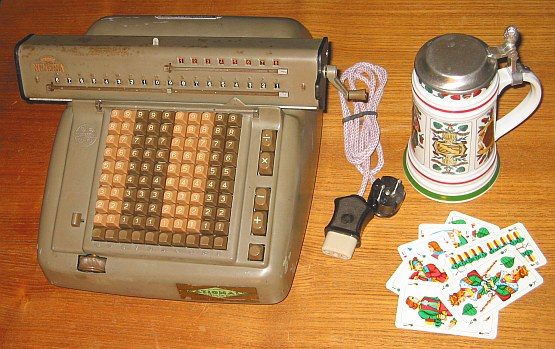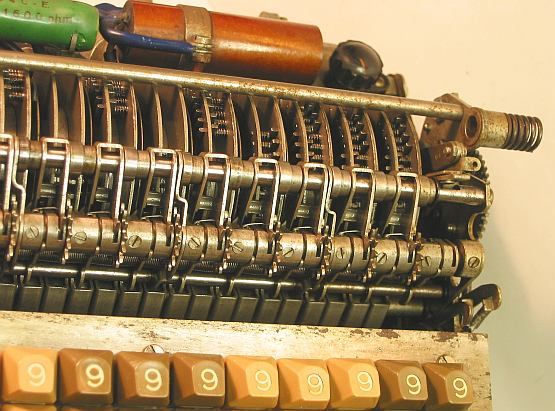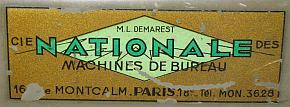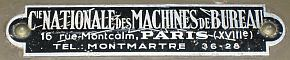previous <<==>> next

NUMERIA 8213
>>>   S P E C I A L  (*)  PINWHEELS w.  A X I A L   SPROCKETS  <<<Have a look at the Restoration Procedure...
 ``` TECHNICAL DATA of the Electro-Mechanical Calculator NUMERIA »8213« *************************************************************************** Serial Number: 050914 Dimensions: (ca.) Width = 14_1/2" / 37 cm Depth = 12_1/2" / 32 cm Height = 6_1/2" / 17 cm Weight: (ca.) 22 lbs / 10 kg Power Supply: 110 / 220 V AC / DC Mechanics: 10*10 Full-Keyboard SPECIAL N U M E R I A CODING (* see below !) ... ... PINWHEELS with A X I A L SPROCKETS Counter Stepping Mechanism + Animation Functions: Add, Subtract, Multiply, Divide Registers: Input = 10 Decimals Counter = 8 Decimals (NO Carry; Neg.Fig.RED) Arithmetic = 18 Decimals Manufacturer: NUMERIA Lagomarsino, Milano / Italy 1965 H O W T O U S E the NUMERIA 8213 **************************************** BEFORE STARTING A TASK: ----------------------- CLEAR the KEYBOARD by pressing the lower right Button. CLEAR the COUNTER unit by turning the carriage crank clockwise. CLEAR the ARITHMETIC unit by turning the same crank counter-clockwise. When the LEVER ON TOP is switched LEFT, THE ARITHMETIC UNIT will N O T BE CLEARED in its LEFT PART (= Positions 18 .. 10 ). Shift the CARRIAGE to the left (= Pos. 1) by turning the wheel in front. GENERAL: -------- Keys [+] & [-] start Add- & Subtract-Procedure and CLEAR KEYBOARD after it. Keys [x] & [:] start "Multiply"- & "Division"-Procedure same as [+] & [-] BUT D O N O T CLEAR KEYBOARD after it. Under COUNTER & ARITHMETIC UNIT are turnable (!) DECIMAL MARKER-BARS: Each bar contains 4 PRE-SET patterns. ADDITION & SUBTRACTION: ----------------------- Example: 123 + 45 - 6 = 162 ADD: Enter the first number (123) in the far right columns. Press the PLUS-key [+] to transfer the number into arithmetic unit. The counting unit displays the figure 1. Enter the second number (45). Press the PLUS- key [+] to add the number. The arithmetic unit displays the intermediate sum (168) and the counting unit displays the figure 2. SUBTRACT: Enter the third number (6). Press the MINUS-key [-]. The arithmetic unit displays the result (162) and the counting unit is decreased by 1. REMARK: NEGATIVE RESULTS are displayed in the arithmetic unit as the COMPLEMENT of the next higher 10, 100, 1000, ... Example: -12 = 99...9988 MULTIPLICATION: --------------- Example: 123 x 45 = 5535 Clear counter and arithmetic units. Enter the multiplicand (123) in the far right columns. The multiplicator (45) has two digits, so the arithmetic unit is moved to position 2 with a clockwise turn of the wheel in front. Repeat pressing the Key [x] until the first figure of the multiplicator (4) will appear in the 2nd position of the counter unit. Move the arithmetic unit to position 1. Repeat pressing Key [x], until the second figure of the multiplicator (5) appears in the 1st position of the counter unit. The multiplication is done: The multiplicand (123) stays in keyboard, the multiplicator (45) is in the counter unit and the result (5535) is in the arithmetic unit. DIVISION: --------- Example: 22 : 7 = 3.1428571 Remainder 3 Division requires 3 steps: (A) To Set the Dividend into Arithmetic Unit: ----------------------------------------- For the maximum number of decimals, pull out the arithmetic unit to the far right position. Enter the dividend (22) in the far right columns. Press the PLUS-key [+] to transfer into arithmetic unit. (B) To Set the Divisor in the Keyboard: ----------------------------------- Clear the counter unit by turning the carriage crank clockwise. Enter the divisor (7) below the dividend (22). (C) To Divide: ---------- Press Key [:] until the arithmetic unit shows an "underflow". Press Key [x] once to correct. Move the carriage to the next left position. Repeat this procedure until the required number of decimals ... The result (3.1428571) is in the counter unit ( in RED ), and the remainder (3) is in the arithmetic unit. The divisor (7) stays in the input unit, therefore an additional decimal can be estimated ... ( 4, cause 4 x 7 = 28). The lower left "ITEM-COUNTER" lever: ------------------------------------ Because the counter has no 10s-carry, there is no "build-in" counting of the items to add. Here the "Item-Counter" lever is useful: When the LEFT KEY  is pressed and the NEIGHBOR-LEFT KEY shifted towards KEY , KEY  is locked. Now the arithmetic unit will count the items in the left column. The "CLEAR KEYBOARD" Button will NOT clear this  key - and when the LEVER ON TOP is switched LEFT, the count will stay when the arithmetic unit will be cleared. Shifting the NEIGHBOR-LEFT KEY left will release Key . Have a look at "Calculating Trickies" ... (*) R E M A R K S : ============================================================================ The COUNTER-FIGURES ( WHITE/RED ) follows the "MONROE-TYPE"-Scheme ... The NUMERIA looks like a MONROE- or NISA-CLONE - BUT I T I S N O T :... PINWHEELS with A X I A L SPROCKETS ! See M.REESE & P.HAERTEL » NUMERIA - nur scheinbar eine MONROE-Tochter « In this 2007 Paper the "DISCOVERY" was made public ... http://www.rechnerlexikon.de/files/NumeriaQuer.pdf There are two S T I C K E R S on the case ( front & back ), refering to an office machine shop in MONTMARTRE / PARIS ...... Wished the calculator could tell his story! PS.: The boring military brown-green appearance of the NUMERIA is contrasted by my SKAT-Mug. The card game "Skat" is popular in Germany like "Fussball". And there is a RELATION: The word "Skat" came from the Italien "scartare" (= "put aside" ) refering to the two cards placed upside-down on the desk. impressum: **************************************************************************** © C.HAMANN http://public.BHT-Berlin.de/hamann 04/17/11 ```